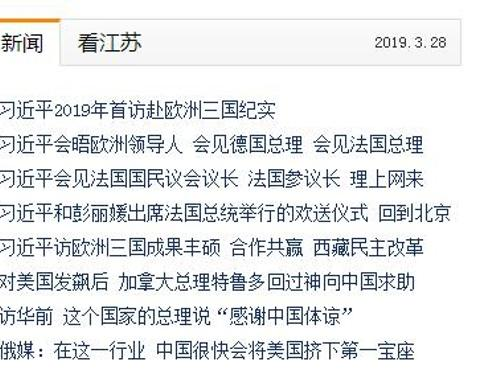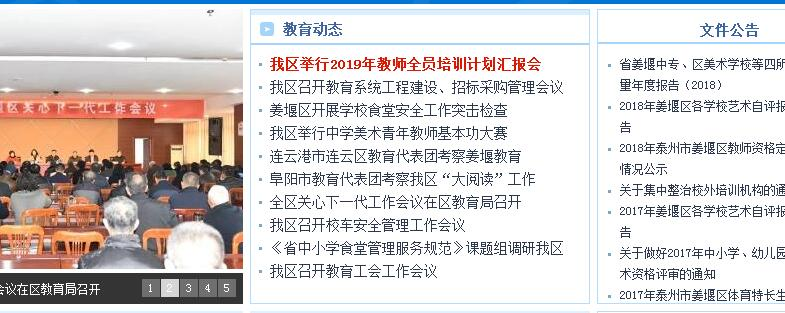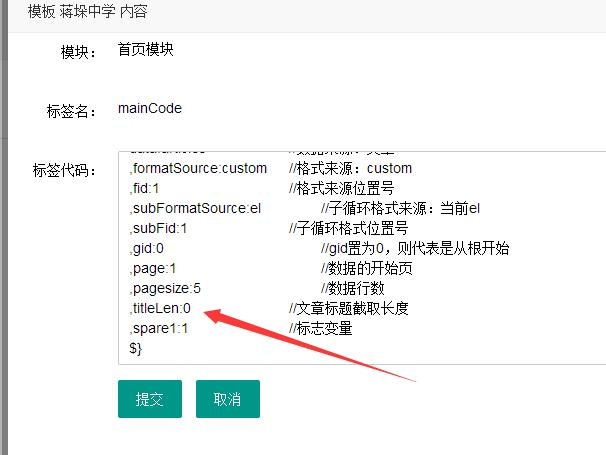2020-03-19 17:12:46<spanhelvetica neue',="" helvetica,="" 'pingfang="" sc',="" 微软雅黑,="" tahoma,="" arial,="" sans-serif;="" font-size:="" 14.4444446563721px;="" font-style:="" normal;="" font-variant:="" font-weight:="" letter-spacing:="" line-height:="" 23.3333339691162px;="" orphans:="" auto;="" text-align:="" left;="" text-indent:="" 0px;="" text-transform:="" none;="" white-space:="" widows:="" word-spacing:="" -webkit-text-stroke-width:="" display:="" inline="" !important;="" float:="" none;"="">我区教师发展中心网站采用jycfd.cn域名

<spanhelvetica neue',="" helvetica,="" 'pingfang="" sc',="" 微软雅黑,="" tahoma,="" arial,="" sans-serif;="" font-size:="" 14.4444446563721px;="" font-style:="" normal;="" font-variant:="" font-weight:="" letter-spacing:="" line-height:="" 23.3333339691162px;="" orphans:="" auto;="" text-align:="" left;="" text-indent:="" 0px;="" text-transform:="" none;="" white-space:="" widows:="" word-spacing:="" -webkit-text-stroke-width:="" display:="" inline="" !important;="" float:="" none;"="">所以，我们在发布文章和模板设计的时候，注意这两个同时运用，会使用网站出现绝佳的呈现效果。